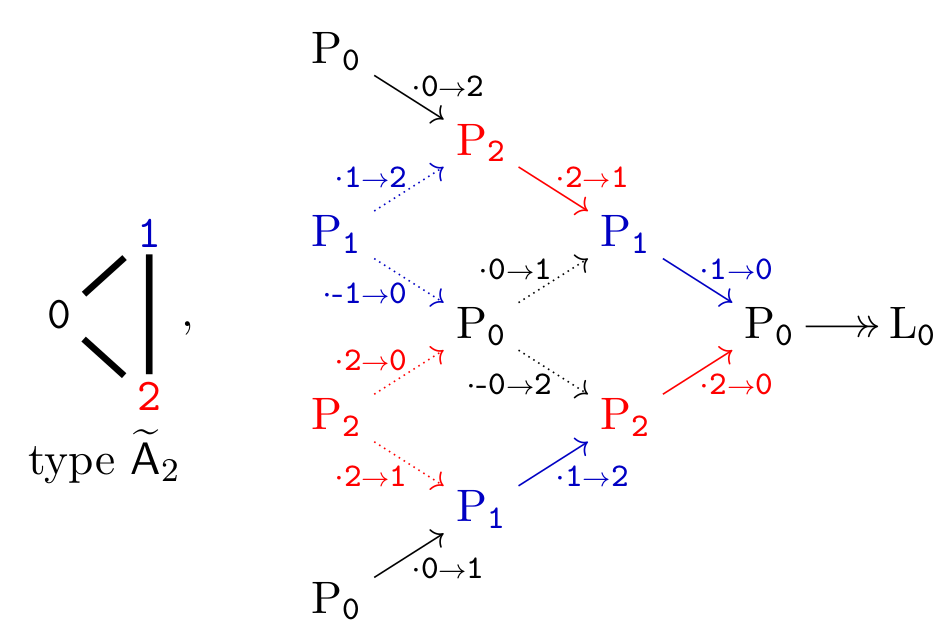## Data

• Title: Algebraic properties of zigzag algebras
• Authors: Michael Ehrig and Daniel Tubbenhauer
• Status: Comm. Algebra 48 (2020), no.1, 11-36. Last update: Tue, 2 Jul 2019 11:28:16 UTC
• ArXiv version = 0.99 published version
• LaTex Beamer presentation: Slides1

## Abstract

We give necessary and sufficient conditions for zigzag algebras and certain generalizations of them to be (relative) cellular, quasi-hereditary or Koszul.

## A few extra words

Let $$\mathrm{Z}_{\rightleftarrows}=\mathrm{Z}_{\rightleftarrows}(\Gamma)$$ be the zigzag algebra associated to a finite, connected, simple graph $$\Gamma$$. The purpose of this note is to show the following.

Theorem A
$$\mathrm{Z}_{\rightleftarrows}$$ is cellular if and only if $$\Gamma$$ is a finite type $$\mathsf{A}$$ graph. $$\mathrm{Z}_{\rightleftarrows}$$ is relative cellular if and only if $$\Gamma$$ is a finite or affine type $$\mathsf{A}$$ graph.
Further, in all cases where $$\mathrm{Z}_{\rightleftarrows}$$ is (relative) cellular, the path length endows it with the structure of a graded (relative) cellular algebra.
Theorem B
$$\mathrm{Z}_{\rightleftarrows}$$ is never quasi-hereditary.
Theorem C
$$\mathrm{Z}_{\rightleftarrows}$$ is Koszul if and only if $$\Gamma$$ is not a type $$\mathsf{ADE}$$ graph.

Moreover, in all cases we construct the corresponding data explicitly.

Let further $$\mathrm{Z}_{\rightleftarrows}^{\mathtt{B}}=\mathrm{Z}_{\rightleftarrows}^{\mathtt{B}}(\Gamma)$$ be the zigzag algebra with a vertex-loop condition (vertex condition for short) set of vertices $$\mathtt{B}\neq\emptyset$$. Using the same ideas as for $$\mathrm{Z}_{\rightleftarrows}$$ we can also prove:

Theorem A$$\mathtt{B}$$
$$\mathrm{Z}_{\rightleftarrows}^{\mathtt{B}}$$ is cellular if and only if $$\Gamma$$ is a finite type $$\mathsf{A}$$ graph and the vertex condition is imposed on one leaf. $$\mathrm{Z}_{\rightleftarrows}^{\mathtt{B}}$$ is relative cellular in exactly the same cases.
Theorem B$$\mathtt{B}$$
$$\mathrm{Z}_{\rightleftarrows}^{\mathtt{B}}$$ is quasi-hereditary if and only if $$\Gamma$$ is a finite type $$\mathsf{A}$$ graph and the vertex condition is imposed on one leaf.
Theorem C$$\mathtt{B}$$
$$\mathrm{Z}_{\rightleftarrows}^{\mathtt{B}}$$ is always Koszul.

Here is an example of how a linear projective resolution might look like:which gives a linear projective resolution of the simple corroding to the vertex 0.